Journal of Statistics Education, V8N2: Paranjpe

# How Many Words in a Dictionary? Innovative Laboratory Teaching of Sampling Techniques

S. A. Paranjpe and Anita Shah
The University of Pune, India

Journal of Statistics Education v.8, n.2 (2000)

Copyright (c) 2000 by S. A. Paranjpe and Anita Shah, all rights reserved. This text may be freely shared among individuals, but it may not be republished in any medium without express written consent from the authors and advance notification of the editor.

Key Words: Active learning; Practicals; Sample surveys; Statistics.

## Abstract

In Indian Universities, courses titled 'Statistics Practical' usually involve only numerical evaluation. There is very little scope for independent thinking and decision making on the part of the students. We report here our experience of teaching a practical course on sampling techniques in a different way. On the whole, it was an encouraging exercise.

# 1. Introduction

1 Reorganizing the contents of a statistics course or changing the methods of teaching a course is a continuous process. Fesco et al. (1996) discuss why the typical course on survey sampling should be restructured and how it can be done to suit the needs of the society. Their discussion concerns a heterogeneous group of students. In a university setting, students in a master's degree course may be a homogeneous group. This article discusses how the current syllabus can be taught using a different approach to provide students with practical experience, helping the material to come alive.

2 In Indian Universities, statistics programs include distribution theory, statistical inference, design of experiments, and sampling theory of surveys as the core courses. The classroom teaching of these courses mostly involves derivation of formulae and proofs of theorems, while numerical examples, if any, are on a Lilliputian scale. The scale is small because large datasets cannot be handled in classrooms, and, until recently, computer facilities were very limited.

3 So-called 'practical' courses in statistics are expected to play a role complementary to the theory classes. The goals of an ideal practical course can be set out as follows:

1. Verifying empirically theoretical results like the central limit theorem and the weak law of large numbers. One can compare this to laboratory verification of known 'laws' in a physics practical.

2. Developing computational skills such as fitting a specified model to a given dataset.

3. Undertaking exploratory analysis in a given situation where the answer is known to the teacher, but unknown to the students.

4. Handling a situation completely unknown to both the teacher and the student and preparing a small project report based on exploration and analysis.

4 At present, probably not many of us go beyond the first two goals listed above. Unfortunately, there is very little scope in the assignments related to goals (1) and (2) for students to think independently. As a result, our practicals become boring and uninteresting. Students just end up plugging numbers into given formulae and writing reject/accept H0 as their 'conclusion' without bothering to think about what H0 is! Hence, alternative ways of conducting the practical courses must be developed. We report here our experience with one such attempt.

5 Any move towards changing this situation requires strong motivation on the part of teachers, reasonable flexibility in the student evaluation system, support from colleagues, and, most importantly, students' cooperation and enthusiasm. We present here our ideas, difficulties encountered by the students, and their responses to a 'practical' on sample surveys. Our M.Sc. program consists of 20 one-semester courses. This study forms one-half of a semester course.

6 Considering the relative weight of this course in the whole program and the time available for this study, a real life problem involving field work was not feasible. Hence a pseudo real life situation was considered.

# 2. The Case Study

## 2.1 Objective

7 In a routine practical course on sample surveys, we assign a different set of questions for each session devoted to a topic, e.g., cluster sampling or simple random sampling without replacement (SRSWOR). Each session is two and a half hours long. The total time spent on the exercise described below was 8 to 10 sessions of two and half hours each over a period of five weeks. In our changed format, we set a common goal for the entire topic of sample surveys. The goal was estimating the total number of words in the Concise Oxford Dictionary (9th edition).

8 The broad objective to be achieved was as follows: Empirical comparisons of different sampling strategies are to be made, and the sampling variance associated with each strategy is to be studied. Standard textbooks on sampling theory like Murthy (1967), Cochran (1977), and Sukhatme, Sukhatme, Sukhatme, and Asok 1984) discuss situations under which one strategy should do better than another. The exercise gives the students a chance to empirically verify these comments. The question set for this exercise is given as Appendix A.

## 2.2 Work Load for Students

9 Each student counted the number of words on 50 pages for each of seven sampling strategies. In addition, for the ratio and regression methods of estimation, the student had to record an auxiliary variable. Hence, a student counted words on 450 pages, a substantial amount of manual work. Thus the students generated data instead of passively receiving it. This gave them an opportunity to appreciate difficulties in data generation.

10 For each sample drawn, the student calculated the mean number of words per page and then estimated the population total and its standard error. The formulae were not provided ready-made. Instead, all prescribed textbooks were made available, and students were asked to find and use the appropriate formulae. This forced them to browse through the books and hence increased the group discussions about notational differences. Further, each student had to draw a different sample. This helped in overcoming one more difficulty. In our usual practical setup where each student has the same question and the same dataset, students are tempted to copy the answers. This was not possible in the modified setup.

## 2.3 Protocol

11 The 'sampling unit' was defined to be one page of the dictionary. Thus pages 1 to 1632 made up the 'sampling frame.' The characteristic under study was a 'word.'

12 The first difficulty we encountered was the definition of 'word.' Various possibilities include the following:

1. Head-word or main-word: Every word that starts at the extreme left of the column on a page. Thus on page number 509, one head-word is 'fist.'

2. Words in bold type: These include head-words and words derived from them like nouns, adjectives, and adverbs. For example, words derived from 'fist' are 'fisted,' 'fistful,' and 'make a good fist.'

3. Words and meanings: The back cover of the dictionary claims that it contains 140 thousand 'meanings' and two million 'texts of words.' As an illustration, we can see that there are three meanings for the word 'fist.' So along with meanings of the derived words, the count by this definition is seven.

13 It was not obvious which definition one should use. Hence a pilot survey of ten pages was done using the first definition, which led to an estimate of 70 thousand words in the dictionary, approximately half the value claimed. When all bold-type words were counted, the estimate rose to 110 thousand. Hence, the second definition was thought to be more appropriate and was adopted for further work. The pilot study also suggested that there could be some relationship between number of head-words and number of bold-type words on a page. This information was useful in illustrating ratio and regression methods of estimation.

## 2.4 Generation of Random Samples

14 The following seven sampling strategies were used in the exercise.

1. SRSWOR: Let R be a random uniform number from U(0,1). Then IR = Int(1632 × R) + 1 is a random integer between 1 and 1632 indicating the page number to be sampled.

2. Stratified Sampling: Natural stratification of the dictionary is by letters of the alphabet, resulting in 26 strata. But some of these strata, namely J, Q, X, Y, and Z, are so small that a sample of size 50 with proportional allocation may never include a page from these strata. Table 1 shows the sample size that would be chosen from each of these strata using a total sample size of 50.

Table 1. Stratum Size and Sample Size for the Five Smallest Strata for Stratified Sampling with a Total Sample Size of 50

 Stratum Stratum size (Ni) Sample size (ni) J 13 0.4 Q 10 0.3 X 2 0.1 Y 6 0.2 Z 4 0.1

Hence, these letters were combined to form one stratum of size 35 with ni = 1, giving a total of 22 strata. Table 2 lists the strata, their sizes, and the corresponding sample sizes. The column of sample sizes contains some entries that are fractional numbers. We can solve this problem by treating each of the two columns on a page as an individual unit. We note that in most cases the observation (i.e., count of words) is recorded only for the whole page. The count is recorded for half pages only when the sample size is fractional. If ni = 1, we cannot estimate the within stratum variation.

The rounding of the sample size was done using the following ad hoc rule. Let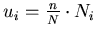be the fractional sample size calculated. Then, if the fractional part of ui is between 0 and 0.25, it is rounded to 0; between 0.25 and 0.75, to .5; and between 0.75 and 1.00, to 1. Using this rule, the estimate of the population total and its standard error required a modification that is discussed in Appendix B.

Table 2. Stratum Sizes and Sample Sizes for Stratified Sampling with a Total Sample Size of 50

 Stratum Stratum size (Ni) Sample size (ni) A 89 2.5 B 91 3 C 156 5 D 88 2.5 E 56 1.5 F 72 2 G 56 2 H 62 2 I 54 1.5 K 18 0.5 L 59 2 M 86 2.5 N 35 1 O 43 1.5 P 138 4 R 83 2.5 S 205 6.5 T 96 3 U 35 1 V 26 1 W 48 1.5 J, Q, X, Y, Z 35 1

3. Cluster Sampling: If the letters of the alphabet are considered as clusters, then some clusters are too large (like C) or too small (like Q). Hence, it was decided to arbitrarily define every set of five consecutive pages to be a cluster. Thus there would be 326 full clusters and one cluster with two pages. Of these, ten clusters were selected using SRSWOR, and words in the chosen sets of five pages were counted.

4. Systematic Sampling: If N denotes the total number of pages and n the sample size, then let K =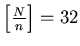. A random digit R is generated between 1 to 32, and the pages numbered R + 32m, m = 0, ..., 49 form the sample. For example, if R is 9, then pages 9, 41, 73, 105, ..., and 1577 form the sample.

5. Two-Stage Sampling: Strata as defined for stratified sampling were considered as first stage units, and five strata were selected using the Midzuno scheme (see Sukhatme et al. 1984). From each of the selected first stage units, ten pages were selected by SRSWOR as second stage units.

6. and 7.   Ratio and Regression Methods: A sample of size fifty was drawn by SRSWOR and head-words (X) and bold-type words (Y) were counted from each page.

## 2.5 Estimation of Standard Errors

15 For the SRSWOR and systematic sampling strategies and the ratio and regression methods of estimation, standard formulae were available in the textbooks. Since fractional sample sizes were used in stratified sampling, the estimator of the population total and its standard error had to be modified suitably. Such small modifications are inevitable when one drifts away from standard problems. Because the derivation of that formula is not the main goal of this article, we give the details in Appendix B.

16 For cluster sampling, multiple clusters were observed, and an ANOVA table could be prepared to obtain estimates of within and between cluster variation. These were then used to estimate the standard error.

17 For two-stage sampling, the formula for the variance of the estimator was not available because the first stage units were drawn by the Midzuno scheme and the second stage units by SRSWOR. Students therefore recognized the merit of adopting an alternative two-stage sampling scheme.

# 3. Results and Discussion

18 The discussion of results below emphasizes the learning process, rather than just reporting the outcome of the sample survey. All students showed interest in the whole exercise. They came up with interesting questions -- statistical and otherwise -- which does not usually happen in our courses. No textbook solutions were available. An example of a question asked was: "On the first page of the dictionary 'A' appears as a bold-type word 16 times. Why should these be treated as 16 separate words?"

19 Such difficulties were solved by group discussions. Hence it became an interactive process. As teachers, we were looking at the study as a whole. This led to some interesting observations that were also discussed in the group.

20 As this was our first experience with the exercise, our involvement was very high. From results submitted by the students, we did a sample check of two pages per strategy per student. Thus we ended up counting words on 280 pages. In the case of the ratio and regression methods, the population total of X (head-words) was needed, which we estimated by counting head-words on about 500 pages. Thus the physical work for the teachers was considerable. But this involvement enabled informed interaction with students regarding the data collection. Based on all the results, students gave a group seminar at the end of the course in which findings based on calculations done by the teachers were also discussed.

21 Table 3 shows a typical summary prepared by a student. In this particular case, cluster sampling had the smallest standard error, and systematic the largest. The others -- namely, SRSWOR, stratified, ratio, and regression -- appear fairly equivalent in terms of empirical precision.

Table 3. Number of Words (× 10-5) Estimated by Different Sampling Schemes and Estimated Standard Errors (× 10-5)

 Sampling schemes.e.95% CI SRSWOR 1.09 0.026 (1.01, 1.11) Stratified 1.08 0.024 (1.04, 1.14) Cluster 1.11 0.007 (1.09, 1.12) Systematic 1.15 0.192 (0.78, 1.53) Two-Stage 1.09 * * Ratio 1.08 0.028 (1.02, 1.13) Regression 1.07 0.022 (1.03, 1.11)

* Standard error and hence CI could not be obtained.

22 Table 4 gives the estimates for the various sampling schemes for all students. From Table 4 the following points can be noted.

• One student (9508) reported extreme estimates. For four strategies, he produced the lowest values, and for systematic sampling, the highest. This unusual circumstance could have been due to an incorrect method of counting words. This occurrence was used as an excuse to discuss the role of non-sampling errors in sample surveys.

• Empirical results indicate that variation across samples is greater for systematic and two-stage sampling than for the other schemes.

• All the estimates were around 100 to 120 thousand. This raises a concern about why all 20 students underestimated the number of words. The question arises whether the bias could be due to the definition of 'word.'

Table 4. Estimates of Total Number of Words (× 10-5) in the Concise Oxford Dictionary

 Student Number SRSWOR Stratified Cluster Systematic Two-Stage Ratio Regression 9403 1.08 1.07 1.05 1.14 1.08 0.99 1.01 9429 1.07 0.95 1.07 1.03 0.97 0.99 1.02 9431 1.03 1.00 1.12 1.14 1.06 1.03 1.08 9432 0.99 1.07 1.10 1.09 1.10 1.09 1.09 9443 1.11 1.07 1.06 1.38 1.11 1.08 1.09 9502 1.04 1.10 0.98 1.33 1.13 1.07 1.08 9503 1.09 1.11 1.08 1.09 1.14 1.07 1.08 9508 0.86 0.99 0.97 1.41 1.05 0.98 0.98 9509 1.10 1.09 1.10 1.12 1.03 1.06 1.08 9510 1.17 1.09 1.10 1.11 1.16 1.07 1.08 9513 0.87 1.04 1.10 1.10 1.15 1.06 1.06 9514 1.10 1.00 1.12 1.09 1.18 1.03 1.03 9517 1.10 1.12 1.09 1.11 1.13 1.04 1.06 9518 1.12 0.98 1.01 1.07 1.11 1.07 1.10 9529 1.02 0.98 1.02 1.07 1.04 0.99 1.01 9532 1.11 1.06 1.12 1.06 1.19 1.08 1.09 9534 1.10 1.06 1.03 1.12 1.11 1.10 1.12 9536 1.07 1.05 1.13 1.07 1.02 1.07 1.12 9540 1.17 1.16 1.13 1.13 1.24 1.11 1.15 9545 1.09 1.08 1.11 1.15 1.09 1.08 1.07 Average 1.06 1.05 1.07 1.14 1.10 1.05 1.07 Empiricalstandard error 0.08 0.05 0.05 0.10 0.06 0.04 0.04 Correctedaverage 1.41 1.40 1.42 1.51 1.47 1.40 1.41

23 To check if the definition of 'word' was the cause of the underestimation, the following exercise was done. This also gave an opportunity to discuss ratio and regression methods. A random sample of 160 pages was drawn by SRSWOR (roughly a 10% sample). Each student counted the number of bold-type words (yi) and number of 'meanings' (wi) on 8 pages. After pooling the data for 160 pages, a correction factor was estimated: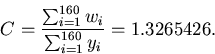All estimates were corrected using this factor to get an estimate of the number of 'meanings.'

24 Averages of these corrected estimates for 20 samples are given in the last row of Table 4. We see that these values (except for systematic and two-stage sampling) are very close to the claim in the dictionary, which means that the third definition of 'word' would have been more appropriate. This helped students realize, somewhat dramatically, how a definitional issue may be far more important than any amount of fine tuning of statistical procedures. Details of how the correction factor may affect the standard error were ignored.

25 In a typical course on sampling theory, we discuss the number of distinct sampling units in a sample drawn with replacement. In the present scheme, individual students sampled without replacement. However, it is possible that the same page could be selected by several students. Hence we thought it would be interesting to ask, "How many distinct pages did the students sample?" We checked this only for the SRSWOR scheme.

26 All students together sampled 20 × 50 = 1000 pages. Of these, 717 were found to be distinct. With n = 50 and N = 1632, the expected number of distinct pages can be obtained as follows:

Probability that a given page will not be selected in a sample of size n is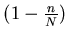.
Probability that a given page will not be selected in any of the 20 samples is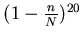.
Expected number of pages not included in any of 20 samples is.
Expected number of pages included in at least one sample is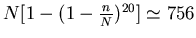. (1)
Variance of number of pages included in at least one sample is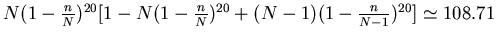. (2)

27 Hence we can test the hypothesis of random selection of pages, using the z test. We obtain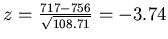. This shows that the observed value is too low. On careful scrutiny, it was suspected that one student might have borrowed observations from other students and put them together. On his own, he counted very few pages.

28 After deleting data from his sample, the observed number of distinct pages for 19 samples turned out to be 705. The expected value 728 and variance 102.94 can be calculated by replacing 20 by 19 in (1) and (2), giving z = -2.27, which is again low. So we still suspect that some students did not choose samples randomly, but rather borrowed some observations from others.

29 Using the distinct pages, the following summary was prepared.

• The minimum number of words on a page was one for page number 89, the last page of the letter A.

• The maximum number of words on a page was 128 for page number 1535 of the letter U.

• Our observation is that, in general, all the pages of the letter U have more words than the pages of other letters. This gives an inflated estimate whenever there is a page from the letter U in a sample. This suggests that pages corresponding to the letter U may have an above average number of words. A possible remedy is to take a sample from the stratum corresponding to the letter U less often than indicated by proportional sampling. Alternatively, we can do a complete enumeration of words starting with the letter U and then sample only from the remaining 25 letters. This illustration was used to discuss the importance of experience and close familiarity with the population being sampled. It became obvious to the class that the use of such knowledge can improve estimates substantially.

• The mean number of words per page was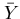= 65.222 with standard deviation 13.795. The lower and upper quartiles were Q1 = 58 and Q3 = 72.

• The frequency distribution of the number of words per page appears symmetric.

• A standard assumption in sample survey theory is that Yi, the characteristic of interest, follows a normal distribution. To verify this assumption, a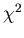goodness of fit test was applied. This showed that fit to the normal was not good. The maximum contribution towas from the modal class. The observed frequency (292) was much larger than the expected frequency (196), indicating more peakedness of the distribution. The lesson learned was the need to be alert about the validity of assumptions. The issue of how to deal with non-normality was not pursued. It is also possible to study the sampling distributions of the statistics computed by the students in a similar manner. However, this was not done in the present exercise.

# 4. Conclusions

• Without the teacher's enthusiasm, such an exercise is difficult.

• Once the teacher takes the initiative, most students do respond positively.

• The boredom of routine computations can be reduced by increasing students' participation in data generation, which was a major component of this practical. However, if the physical work involved is too heavy, the assignment can be shared by a pair or group of students, as was done for the correction factor calculations.

• The implementation of such an exercise is most effective if the class size is small, say, ten.

• We feel that classroom teaching should be supported by activities of this type wherever possible. The benefit of such activities is illustrated by this student comment: "This is the first time that I have understood what a stratum is and how it arises in a practical situation."

• This approach can be problematic if we are exploring a totally unfamiliar situation. We may have to face many difficulties for which textbook solutions may not be available. We must be open to discussions and subsequent changes.

• Such activities give the students a chance to handle at least a pseudo real life situation.

• Other real life problems that can be taken up in a similar way include

• Estimating the number of telephone subscribers in a city using a directory.

• Estimating the total capital investment for industries included in a stock exchange directory.

• Estimating the sex ratio using an electoral roll.

• The conduct of examinations was no more difficult than routine examinations. A data file containing page numbers and corresponding numbers of words was prepared using information on distinct pages. This was treated as the population. Each student was asked to draw two samples -- one by SRSWOR and the other by one of the remaining strategies. Students were asked to estimate the population total and its standard error and to compare the two strategies. The fact that we knew the true value helped in assessment.

## Acknowledgments

We wish to express our sincere thanks to Drs. A. P. Gore, A. V. Kharshikar, and M. B. Rajarshi for help and encouragement throughout the work. We also thank the referees for their valuable comments which helped in improving this material.

# Appendix A: Assignment for the Exercise

Aim: To estimate the number of words in the dictionary. We will study the following aspects of sampling theory:

• For a given sampling strategy, how to estimate the standard error of the estimate.

• How to compare the estimates given by different strategies.

• To what extent principles, assumptions, and theoretical results are applicable in a given practical situation.

Observations and calculations: Use each sampling strategy below to draw 50 random integers between 1 and 1632; these are the page numbers to be sampled. Count the number of words in bold type on each page.

1. List the random digit (i) and the number of words (yi).

2. Estimate the total number of words.

3. Estimate the standard error of your estimate.

4. Obtain 95% confidence intervals for your estimates.

5. Collect estimates from all others.

6. Estimate the standard error of your estimates empirically.

Carry out (a) to (f) for each of the following sampling strategies:

1. Simple Random Sampling Without Replacement
Use (i) the standard method of estimation, (ii) the ratio method, and (iii) the regression method. For (ii) and (iii), the auxiliary information X is the number of head-words on a page.

2. Stratified Random Sampling (standard method of estimation)

3. Cluster Sampling (standard method of estimation)
Treat five consecutive pages as a cluster and draw a sample of ten clusters.

4. Two-Stage Sampling (standard method of estimation)
Draw a sample of five strata out of 22, with probability proportional to stratum size. From each letter chosen, draw a sample of size ten using SRSWOR.

5. Systematic Sampling (standard method of estimation)
Suppose N is the total number of pages in the dictionary. Let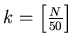. Select a random number between 1 and k and draw a systematic sample of size 50.

# Appendix B: Stratified Sampling -- Modification for Fractional Sample Size

The formulae derived below apply to a single sample of fractional sample size chosen from the N = 1632 pages in the dictionary. In stratified sampling, these formulae are applied to each stratum, and N refers to the size of the stratum. An estimated total and its variance are computed for each stratum. To estimate the population total, one must take the weighted sum of stratum means with the stratum sizes as the weights. The estimate of the standard error will have two components, one for strata with integer sample sizes (derived using textbook formulae) and one for strata with fractional sample sizes (derived using the modified formulae given below).

Let Y1, ..., Yn, Y(h)n+1 be a sample with fractional sample size, i.e., Y1, ..., Yn are observations on n full units, and Y(h)n+1 denotes an observation on a half unit, i.e., one of the two columns on the (n+1)th page. Let Z1 and Z2 represent observations on the two columns of the first unit, Z3 and Z4 observations on the two columns of the second unit, etc. Then, Z2m-1 and Z2m are observations on the two columns of the nth unit, and Z2m+1 = Y(h)n+1. We do not observe Z1 and Z2 separately, but rather Y1 = Z1 + Z2, Y2 = Z3 + Z4, etc.

Assume Z1, Z2, ..., Z2m+1 are a random sample from a normal distribution with mean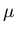and variance. Then the population total is given by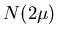, where N = 1632. Consider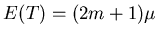, and hence=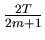is an unbiased estimator of.

To obtain an estimator of var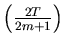, considerThen,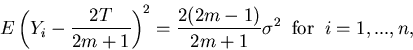and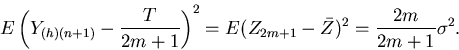Define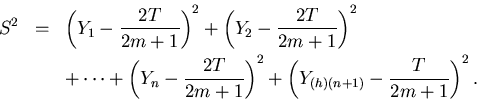Then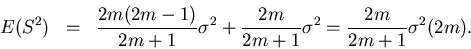It follows that S2/m2 is an unbiased estimator of var= var() =.

# References

Cochran, W. G. (1977), Sampling Techniques (3rd ed.), New York: John Wiley and Sons.

Fesco, R. S., Kalsbeek, W. D., Lohr, S. L., Scheaffer, R. L., Scheuren, F. J., and Stasny, E. A. (1996), "Teaching Survey Sampling," The American Statistician, 50, 328-340.

Murthy, M. N. (1967), Sampling Theory and Methods, Calcutta: Statistical Publishing Society.

Sukhatme, P. V., Sukhatme, B. V., Sukhatme, S., and Asok C. (1984), Sampling Theory of Surveys with Applications, Iowa State University Press and Indian Society of Agricultural Statistics.

S. A. Paranjpe       saparanj@stats.unipune.ernet.in
Anita Shah           shah_anita@yahoo.com
Department of Statistics
The University of Pune
Pune - 411007
Maharashtra, India

JSE Homepage | Subscription Information | Current Issue | JSE Archive (1993-1998) | Data Archive | Index | Search JSE | JSE Information Service | Editorial Board | Information for Authors | Contact JSE | ASA Publications